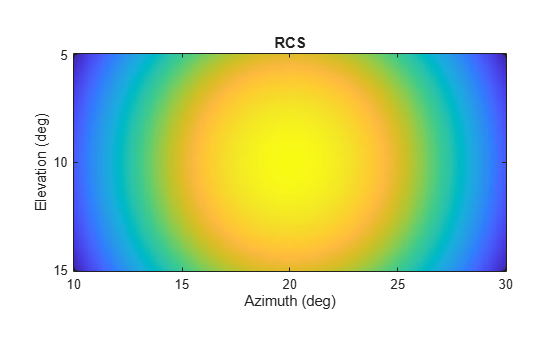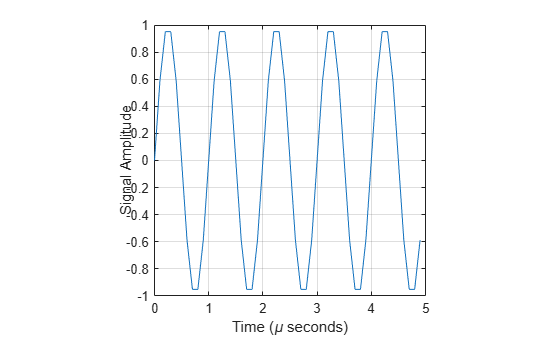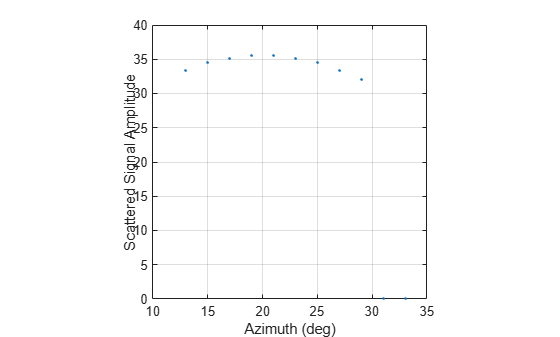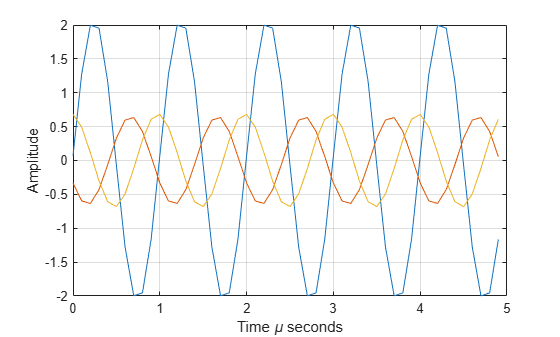## Description

The phased.BackscatterRadarTarget System object™ models the backscattering of a signal from a target. Backscattering is a special case of radar target scattering when the incident and reflected angles are the same. This type of scattering applies to monostatic radar configurations. The radar cross-section determines the backscattering response of a target to an incoming signal. This System object lets you specify an angle-dependent radar cross-section model that covers a range of incident angles.

The phased.BackscatterRadarTarget System object creates a backscattered signal for polarized and nonpolarized signals. While electromagnetic radar signals are polarized, you can often ignore polarization in your simulation and process the signals as scalar signals. To ignore polarization, specify the EnablePolarization property as false. To employ polarization, specify the EnablePolarization property as true.

For nonpolarized signals, you specify the radar cross section as an array of radar cross-section (RCS) values at discrete azimuth and elevation points. The System object interpolates values for incident angles between array points. For polarized signals, you specify the radar scattering matrix using three arrays defined at discrete azimuth and elevation points. These three arrays correspond to the HH, HV, and VV polarization components. The VH component is computed from the conjugate symmetry of the HV component.

For both nonpolarized and polarized signal cases, you can employ one of four Swerling models to generate random fluctuations in the RCS or radar scattering matrix. Choose the model using the Model property. Then, use the SeedSource and Seed properties to control the fluctuations.

EnablePolarizationUse these properties
false

RCSPattern

trueShhPattern, SvvPattern, and ShvPattern

To model a backscattered radar signal:

1. Define and set up your radar target. You can set phased.BackscatterRadarTarget System object properties at construction time or leave them to their default values. See Construction. Some properties that you set at construction time can be changed later. These properties are tunable.

2. To compute the reflected signal, call the step method of phased.BackscatterRadarTarget. The output of the method depends on the properties of the phased.BackscatterRadarTarget System object. You can change tunable properties at any time.

Note

Starting in R2016b, instead of using the step method to perform the operation defined by the System object, you can call the object with arguments, as if it were a function. For example, y = step(obj,x) and y = obj(x) perform equivalent operations.

## Construction

target = phased.BackscatterRadarTarget creates a backscatter radar target System object, target.

target = phased.BackscatterRadarTarget(Name,Value) creates a backscatter radar target System object, target, with each specified property Name set to the specified Value. You can specify additional name and value pair arguments in any order as (Name1,Value1,...,NameN,ValueN).

## Properties

expand all

Option to enable processing of polarized signals, specified as false or true. Set this property to true to allow the target to simulate the reflection of polarized radiation. Set this property to false to ignore polarization.

Example: true

Data Types: logical

Azimuth angles used to define the angular coordinates of each column of the matrices specified by the RCSPattern, ShhPattern, ShvPattern, or SvvPattern properties. Specify the azimuth angles as a length P vector. P must be greater than two. Angle units are in degrees.

Example: [-45:0.1:45]

Data Types: double

Elevation angles used to define the angular coordinates of each row of the matrices specified by the RCSPattern, ShhPattern, ShvPattern, or SvvPattern properties. Specify the elevation angles as a length Q vector. Q must be greater than two. Angle units are in degrees.

Example: [-30:0.1:30]

Data Types: double

Radar cross-section (RCS) pattern, specified as a Q-by-P real-valued matrix or a Q-by-P-by-M real-valued array. Q is the length of the vector in the ElevationAngles property. P is the length of the vector in the AzimuthAngles property. M is the number of target patterns. The number of patterns corresponds to the number of signals passed into the step method. You can, however, use a single pattern to model multiple signals reflecting from a single target. Pattern units are square-meters.

You can also specify the pattern as a function only of azimuth for a single elevation. In this case, specify the pattern as either a 1-by-P vector or an M-by-P matrix. Each row is a separate pattern.

This property applies when the EnablePolarization property is false.

Example: [1,.5;.5,1]

Data Types: double

Radar scattering matrix HH polarization component, specified as a Q-by-P complex-valued matrix or a Q-by-P-by-M complex-valued array. Q is the length of the vector in the ElevationAngles property. P is the length of the vector in the AzimuthAngles property. M is the number of target patterns. The number of patterns corresponds to the number of signals passed into the step method. You can, however, use a single pattern to model multiple signals reflecting from a single target. Scattering matrix units are meters.

You can also specify the pattern as a function only of azimuth for a single elevation. Then, specify the pattern as either a 1-by-P vector or an M-by-P matrix. Each row is a separate pattern.

This property applies when the EnablePolarization property is true.

Example: [1,1;1i,1i]

Data Types: double
Complex Number Support: Yes

Radar scattering matrix VV polarization component, specified as a Q-by-P complex-valued matrix or a Q-by-P-by-M complex-valued array. Q is the length of the vector in the ElevationAngles property. P is the length of the vector in the AzimuthAngles property. M is the number of target patterns. The number of patterns corresponds to the number of signals passed into the step method. You can, however, use a single pattern to model multiple signals reflecting from a single target. Scattering matrix units are meters.

You can also specify the pattern as a function only of azimuth for a single elevation. In this case, specify the pattern as either a 1-by-P vector or an M-by-P matrix. Each row is a separate pattern.

This property applies when the EnablePolarization property is true.

Example: [1,1;1i,1i]

Data Types: double
Complex Number Support: Yes

Radar scattering matrix HV polarization component, specified as a Q-by-P complex-valued matrix or a Q-by-P-by-M complex-valued array. Q is the length of the vector in the ElevationAngles property. P is the length of the vector in the AzimuthAngles property. M is the number of target patterns. The number of patterns corresponds to the number of signals passed into the step method. You can, however, use a single pattern to model multiple signals reflecting from a single target. Scattering matrix units are meters.

You can also specify the pattern as a function only of azimuth for a single elevation. In this case, specify the pattern as either a 1-by-P vector or an M-by-P matrix. Each row is a separate pattern.

This property applies when the EnablePolarization property is true.

Example: [1,1;1i,1i]

Data Types: double
Complex Number Support: Yes

Target fluctuation model, specified as 'Nonfluctuating', 'Swerling1', 'Swerling2', 'Swerling3', or 'Swerling4'. If you set this property to a value other than 'Nonfluctuating', use the update input argument when calling step.

Example: 'Swerling3'

Data Types: char

Signal propagation speed, specified as a positive scalar. Units are in meters per second. The default propagation speed is the value returned by physconst('LightSpeed'). See physconst for more information.

Example: 3e8

Data Types: double

Operating frequency, specified as a positive scalar. Units are in Hz.

Example: 1e9

Data Types: double

Seed source of random number generator for RCS fluctuation model, specified as 'Auto' or 'Property'. When you set this property to 'Auto', the System object generates random numbers using the default MATLAB® random number generator. When you set this property to 'Property', you specify the random number generator seed using the Seed property. This property applies when you set the Model property to'Swerling1', 'Swerling2', 'Swerling3', or 'Swerling4'. When you use this object with Parallel Computing Toolbox™ software, you set this property to 'Auto'.

Example: 'Property'

Data Types: char

Random number generator seed, specified as a nonnegative integer less than 232. This property applies when the SeedSource property is set to 'Property'.

Example: 32301

Data Types: double

## Methods

 reset Reset states of System object step Backscatter incoming signal
Common to All System Objects
release

Allow System object property value changes

## Examples

collapse all

Calculate the reflected radar signal from a nonfluctuating point target with a peak RCS of 10.0 ${m}^{2}$. Use a simplified expression of an RCS pattern of a target for illustrative purposes. Real RCS patterns are more complicated. The RCS pattern covers a range of angles from 10°–30° in azimuth and 5°–15° in elevation. The RCS peaks at 20° azimuth and 10° elevation. Assume that the radar operating frequency is 1 GHz and that the signal is a sinusoid at 1 MHz.

Note: This example runs only in R2016b or later. If you are using an earlier release, replace each call to the function with the equivalent step syntax. For example, replace myObject(x) with step(myObject,x).

Create and plot the RCS pattern.

azmax = 20.0; elmax = 10.0; azpatangs = [10.0:0.1:30.0]; elpatangs = [5.0:0.1:15.0]; rcspattern = 10.0*cosd(4*(elpatangs - elmax))'*cosd(4*(azpatangs - azmax)); imagesc(azpatangs,elpatangs,rcspattern) axis image axis tight title('RCS') xlabel('Azimuth (deg)') ylabel('Elevation (deg)')Generate and plot 50 samples of the radar signal.

foper = 1.0e9; freq = 1.0e6; fs = 10*freq; nsamp = 50; t = [0:(nsamp-1)]'/fs; sig = sin(2*pi*freq*t); plot(t*1e6,sig) xlabel('Time (\mu seconds)') ylabel('Signal Amplitude') gridCreate the phased.BackscatterRadarTarget System object™.

target = phased.BackscatterRadarTarget('Model','Nonfluctuating',... 'AzimuthAngles',azpatangs,'ElevationAngles',elpatangs,... 'RCSPattern',rcspattern,'OperatingFrequency',foper);

For a sequence of incident angles at constant elevation angle, find and plot the scattered signal amplitude.

az0 = 13.0; el = 10.0; naz = 20; az = az0 + [0:2:20]; naz = length(az); ss = zeros(1,naz); for k = 1:naz y = target(sig,[az(k);el]); ss(k) = max(abs(y)); end plot(az,ss,'.') xlabel('Azimuth (deg)') ylabel('Scattered Signal Amplitude') gridCalculate the polarized radar signal scattered from a Swerling1 fluctuating point target. Assume the target axis is rotated from the global coordinate system. Use simple expressions for the scattering patterns for illustration. Real scattering patterns are more complicated. For polarized signals, you need to specify the HH, HV, and VV components of the scattering matrix for a range of incident angles. In this example, the patterns cover the range 10°–30° in azimuth and 5°–15° in elevation. Angles are with respect to the target local coordinate system. Assume that the radar operating frequency is 1 GHz and that the signal is a sinusoid with a frequency of 1 MHz. The incident angle is 13.0° azimuth and 14.0° elevation with respect to the target orientation.

Note: This example runs only in R2016b or later. If you are using an earlier release, replace each call to the function with the equivalent step syntax. For example, replace myObject(x) with step(myObject,x).

Create and plot the scattering matrix patterns.

azmax = 20.0; elmax = 10.0; azpatangs = [10.0:0.1:35.0]; elpatangs = [5.0:0.1:15.0]; shhpat = cosd(4*(elpatangs - elmax))'*cosd(4*(azpatangs - azmax)); shvpat = 1i*cosd(4*(elpatangs - elmax))'*sind(4*(azpatangs - azmax)); svvpat = sind(4*(elpatangs - elmax))'*sind(4*(azpatangs - azmax)); subplot(1,3,1) imagesc(azpatangs,elpatangs,abs(shhpat)) axis image axis tight title('HH') xlabel('Azimuth (deg)') ylabel('Elevation (deg)') subplot(1,3,2) imagesc(azpatangs,elpatangs,abs(shvpat)) axis image axis tight title('HV') xlabel('Azimuth (deg)') subplot(1,3,3) imagesc(azpatangs,elpatangs,abs(svvpat)) axis image axis tight title('VV') xlabel('Azimuth (deg)')Create the phased.BackscatterRadarTarget System object™.

target = phased.BackscatterRadarTarget('EnablePolarization',true,... 'Model','Swerling1','AzimuthAngles',azpatangs,... 'ElevationAngles',elpatangs,'ShhPattern',shhpat,'ShvPattern',shvpat,... 'SvvPattern',svvpat);

Generate 50 samples of a polarized radar signal.

foper = 1.0e9; freq = 1.0e6; fs = 10*freq; nsamp = 50; t = [0:(nsamp-1)]'/fs; signal.X = exp(1i*2*pi*freq*t); signal.Y = exp(1i*2*pi*freq*t + pi/3); signal.Z = zeros(size(signal.X)); tgtaxes = azelaxes(60,10); ang = [13.0;14.0];

Reflect the signal from the target and plot its components.

refl_signal = target(signal,ang,tgtaxes,true); figure plot(t*1e6,real(refl_signal.X)) hold on plot(t*1e6,real(refl_signal.Y)) plot(t*1e6,real(refl_signal.Z)) hold off xlabel('Time \mu seconds') ylabel('Amplitude') gridexpand all

## References

 Mott, H. Antennas for Radar and Communications. New York: John Wiley & Sons, 1992.

 Richards, M. A. Fundamentals of Radar Signal Processing. New York: McGraw-Hill, 2005.

 Skolnik, M. Introduction to Radar Systems, 3rd Ed. New York: McGraw-Hill, 2001.